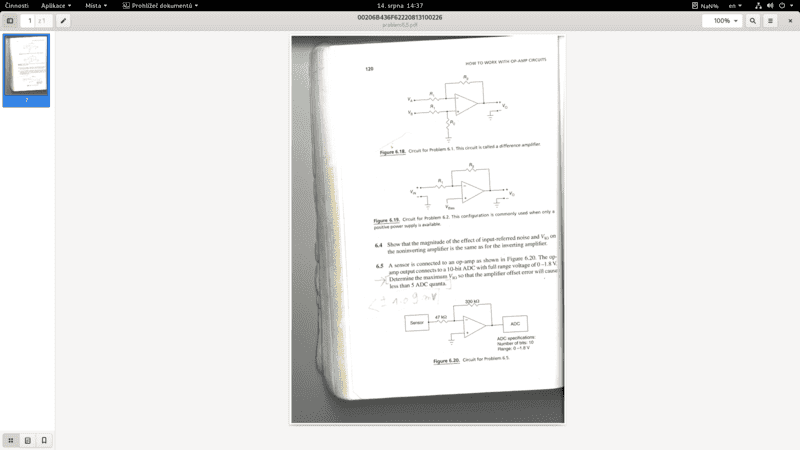# Maximum input offset voltage

• Engineering
• electronic engineer

#### electronic engineer

Substandard Post Quality
Homework Statement
Relevant Equations
V(o)=-V(io)*(1+R2/R1)
Hello,

i have questions from a book that I'm reading, it's full of interesting problems about Electrical Engineering. For now i want to ask about op-amp offset error.

I will post a copy of the problem here.

We can talk about it, i have an idea about offset error so i can share it here later, but for now can you just tell me about ADC quanta?Thank you.

Homework Statement:: op-amp with 10-bit ADC
Relevant Equations:: V(o)=-V(io)*(1+R2/R1)

We can talk about it, i have an idea about offset error so i can share it here later, but for now can you just tell me about ADC quanta?
•